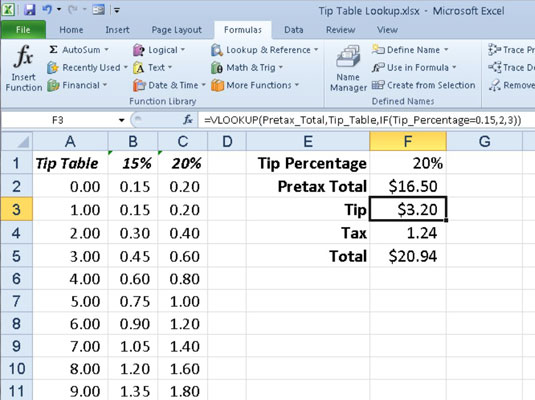##### Excel 2010 All-in-One For DummiesThe most popular of the lookup functions in Excel 2010 are HLOOKUP (for Horizontal Lookup) and VLOOKUP (for Vertical Lookup). These functions are located in the Lookup & Reference category on the Formulas tab of the Ribbon and in the Insert Function dialog box. They are part of a powerful group of functions that can return values by looking them up in data tables.

The HLOOKUP function searches horizontally (left to right) the topmost row of a Lookup table until it locates a value that matches or nearly matches the one that you're looking up. The VLOOKUP function searches vertically (top to bottom) the leftmost column of a Lookup table until it locates a value that matches or nearly matches the one you're looking up.

When using the VLOOKUP and HLOOKUP functions, the text or numeric entries in the Lookup column or row (that is, the leftmost column of a vertical Lookup table or the top row of a horizontal Lookup table) must be unique. These entries must also be arranged or sorted in ascending order; that is, alphabetical order for text entries, and lowest-to-highest order for numeric entries.

The HLOOKUP function uses the following syntax:

`=HLOOKUP(lookup_value,table_array,row_index_num,[range_lookup])`

The VLOOKUP function follows the nearly identical syntax:

`=VLOOKUP(lookup_value,table_array,col_index_num,[range_lookup])`

In both functions, the lookup_value argument is the value that you want to look up in the table, and table_array is the cell range or name of the table that contains both the value to look up and the related value to return. The row_index_num argument in the HLOOKUP function is the number of the row whose value you want to return; the col_index_num argument in the VLOOKUP function is the number of the column whose value you want to return.

The optional range_lookup argument in both the VLOOKUP and the HLOOKUP functions is the logical TRUE or FALSE that specifies whether you want Excel to find an exact or approximate match for the lookup_value in the table_array. When you specify TRUE or omit the range_lookup argument, Excel finds an approximate match. When you specify FALSE as the range_lookup argument, Excel finds only exact matches.

Finding approximate matches pertains only to when you're looking up numeric entries (rather than text). When Excel doesn't find an exact match in this Lookup column or row, it locates the next highest value that doesn't exceed the lookup_value argument and then returns the value in the column or row designated by the col_index_num or row_index_num arguments.

The figure below shows an example of using the VLOOKUP function to return either a 15% or 20% tip from a tip table. Cell F3 contains the VLOOKUP function:

`=VLOOKUP(Pretax_Total,Tip_Table,IF(Tip_Percentage=0.15,2,3))`

This formula returns the amount of the tip based on the tip percentage in cell F1 and the pretax amount of the check in cell F2.Using the VLOOKUP function to return the amount of the tip to add from a Lookup table.

To use this tip table, enter the percentage of the tip (15% or 20%) in cell F1 (named Tip_Percentage) and the amount of the check before tax in cell F2 (named Pretax_Total). Excel then looks up the value that you enter in the Pretax_Total cell in the Lookup table, which includes the cell range A2:C101 and is named Tip_Table.

Excel then moves down the values in the first column of Tip_Table until it finds a match. If Excel finds that the value entered in the Pretax_Total cell (\$16.50 in this example) doesn't exactly match one of the values in the first column of Tip_Table, the program continues to search down the comparison range until it encounters the first value that exceeds the pretax total (17.00 in cell A19 in this example). Excel then moves back up to the previous row in the table and returns the value in the column that matches the col_index_num argument of the VLOOKUP function (this is because the optional range_lookup argument has been omitted from the function).

Notice that the col_index_num argument uses an IF statement to determine which column's value to return. In this case, if the Tip_Percentage value is 0.15, then the function returns the value in the second column of the table. Otherwise, it returns the value in the third column of the same row.